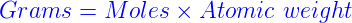# Moles to Grams Formula

Mole is the standard unit of amount measurement. Substances react in simple ratios of moles. But, when you have to compare the amount of one substance to another by moles, you must convert into grams since balances do not provide readings in moles.

You have three steps to convert mole values to grams.

1.    Calculate how many moles are mentioned in the question.
2.    Find the molar mass of the substance.
3.    Multiply both the values.

One mole consists of Avogadro number of atoms. If you know the quantity of mole, it can be converted into grams and vice versa.

The formula for moles to grams is given byExample 1Calculate the mass in grams of 3.6 mol of H2SO4.

Solution

Look for the atomic masses of hydrogen, sulfur and oxygen.

H = 1.008

S = 32.06

O = 16

Therefore, the molecular mass of H2SO4 is

2(1.008) + 32.06 + 4(18) = 106.076

Hence, one mole of H2SO4 weights 106.076 grams.

Since you need to find for 3.60 mol of H2SO4

= 3.60 mol x 106.076  g/mol

= 381.87 g of H2SO4

Example 2.

Convert 3 moles of carbon monoxide to grams.

Solution

Find the molecular weight of Carbon monoxide.

By calculating, we get the molecular weight of CO = 28.01 g/mol

Since we need to find for 3 moles of Carbon monoxide

= 3 x 28.01 = 84.03 grams